## Friday, 8 August 2014

### CHAPTER 6 - Multiplication of a Vector by a Scalar

Intuitively, we can expect that if we multiply a vector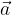$\vec a$ by some scalar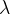$\lambda$, the support of the vector will not change; only its magnitude and / or its sense will. Specifically, if$\lambda$ is positive, the vector will have the same direction; only its length will get scaled according to the magnitude of$\lambda$. If$\lambda$ is negative, the direction of the product vector will be opposite to that of the original vector; the length of the product vector will depend on the magnitude of$\lambda$.Note that for any vector$\vec a$, if we denote the unit vector along$\vec a$ by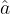$\hat a$, we have${\vec a = \left| {\,\vec a\,} \right|\hat a}$
Put in words, if we multiply the unit vector along a vector$\vec a$ by its magnitude, we obtain that vector itself. Put in a slightly different way, we have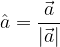$\hat a = \dfrac{{\vec a}}{{\left| {\vec a} \right|}}$
i.e, if we divide a vector by its magnitude, we obtain the unit vector along that vector’s direction.
Another very important result that follows from this discussion is that two vectors$\vec a$ and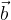$\vec b$ are collinear if and only if there exists some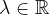$\lambda \in \mathbb{R}$ such that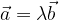${\vec a = \lambda \vec b}$
Collinear vectors
i.e, two vectors are collinear if one can be obtained from the other simply by multiplying the latter with a scalar.
This fact can be stated in another way : consider two non-collinear vectors$\vec a$and$\vec b$. If for some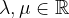$\lambda ,\mu \in \mathbb{R}$, the relation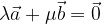$\lambda \vec a + \mu \vec b = \vec 0$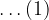$\ldots(1)$
is satisfied, then$\lambda$ and$\mu$ must be zero. This is because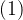$(1)$ can be written as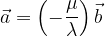$\vec a = \left( { - \dfrac{\mu }{\lambda }} \right)\vec b$
which would imply that$\vec a$ is a scalar multiple of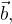$\vec b,$ i.e.$\vec a$ and$\vec b$, are collinear, contradicting our initial supposition that$\vec a$ and$\vec b$ are non-collinear.
In subsequent discussions, we’ll be talking a lot about linear combinations of vectors. Let us see what we mean by this. Consider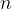$n$ arbitrary vectors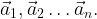${\vec a_1},{\vec a_2}\ldots {\vec a_n}.$ A linear combination of these$n$ vectors is a vector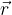$\vec r$ such that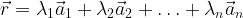$\vec r = {\lambda _1}{\vec a_1} + {\lambda _2}{\vec a_2} + \ldots + {\lambda _n}{\vec a_n}$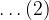$\ldots(2)$
where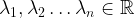${\lambda _1},{\lambda _2}\ldots {\lambda _n} \in\mathbb{R}$ are arbitrary scalars. Any sort of combination of the form in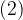$(2)$ will be termed a linear combination.
Thus, using the terminology of linear combinations, we can restate the result we obtained earlier: for any two non-zero and non-collinear vectors$\vec a$ and$\vec b$, if their linear combination is zero, then both the scalars in the linear combination must be zero.
We now come to a very important concept.

#### THE BASIS OF A VECTOR SPACE

Consider a two-dimensional plane, and any two arbitrary non-collinear vectors$\vec a$ and$\vec b$ in this plane. We make the two vectors co-initial and use their supports as our reference axes:Observe carefully that any vector$\vec r$ in the plane can be represented in terms of$\vec a$ and$\vec b$. We find the components of$\vec r$ along the directions of$\vec a$ and$\vec b$; those components must be some scalar multiples of$\vec a$ and$\vec b$.Thus, any vector$\vec r$ in the plane can be written as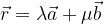$\vec r = \lambda \vec a + \mu \vec b$ for some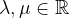$\lambda ,\mu \in\mathbb{R}$$\ldots(1)$
i.e, any vector$\vec r$ in the plane can be expressed as a linear combination of$\vec a$ and$\vec b$.
We state this fact in mathematical terms as follows: the vectors$\vec a$ and$\vec b$ form a basis of our vector space (which is a plane in this case). The term “basis” means that using only$\vec a$ and$\vec b$, we can construct any vector lying in the plane of$\vec a$ and$\vec b$.
Note that there’s nothing special about$\vec a$ and$\vec b$; any two non-collinear vectors can form a basis for the plane.
You must be very clear on the point that two collinear vectors cannot form the basis for a plane while any two non-collinear vectors can. Understanding this fact is very crucial to later discussions.
Try proving this: let$\vec a$ and$\vec b$ form the basis of a plane. For any vector$\vec r$ in the plane of$\vec a$ and$\vec b$, we can find scalars$\lambda ,\mu \in\mathbb{R}$ such that$\vec r = \lambda \vec a + \mu \vec b$
Prove that this representation is unique
The basic principle that we’ve learnt in this discussion can be expressed in a very useful way as follows:
Three vectors are coplanar if and only if one of them can be expressed as a linear combination of the other two. i.e., three vectors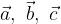$\vec a,\,\,\vec b,\,\,\vec c$ are coplanar if there exist scalars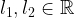${l_1},{l_2} \in\mathbb{R}$ such that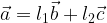$\vec a = {l_1}\vec b + {l_2}\vec c$
We can write this as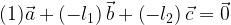$(1) \vec a + \left( { - {l_1}} \right)\vec b + \left( { - {l_2}} \right)\vec c = \vec 0$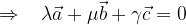$\Rightarrow \,\,\,\,\, \lambda \vec a + \mu \vec b + \gamma \vec c = 0$
This form equivalently tells us that three vectors are coplanar if we can find three scalars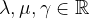$\lambda ,\mu ,\gamma \in \mathbb{R}$ for which their linear combination is zero.